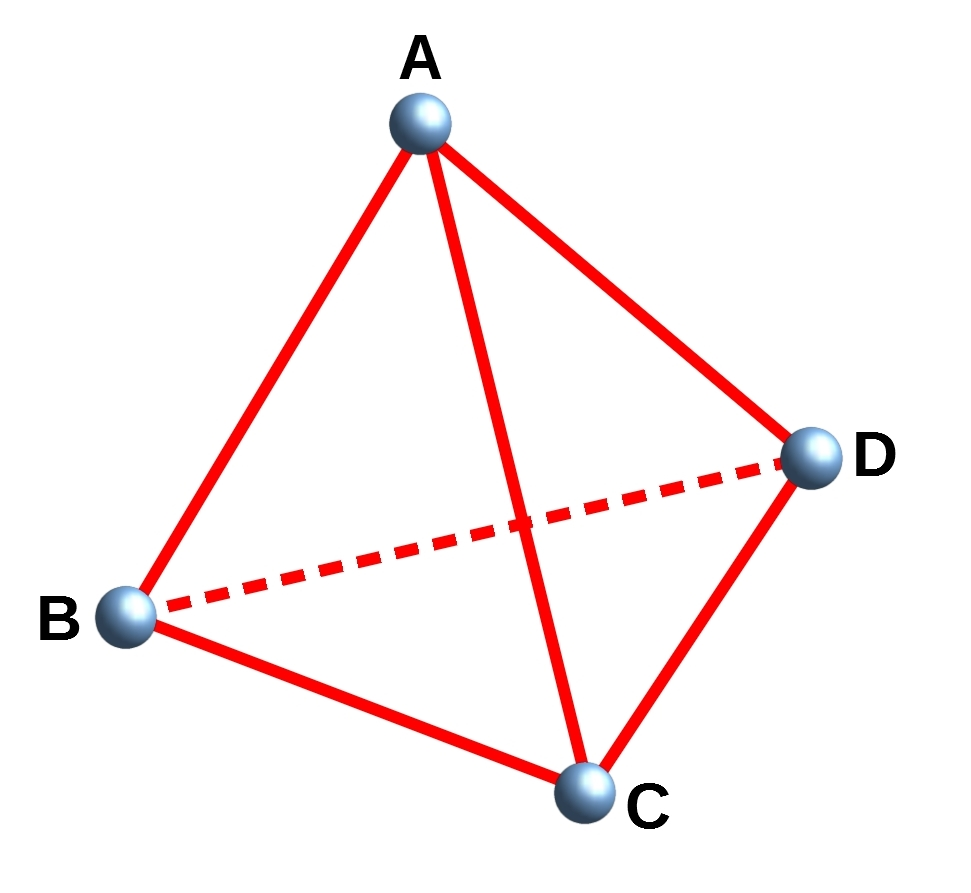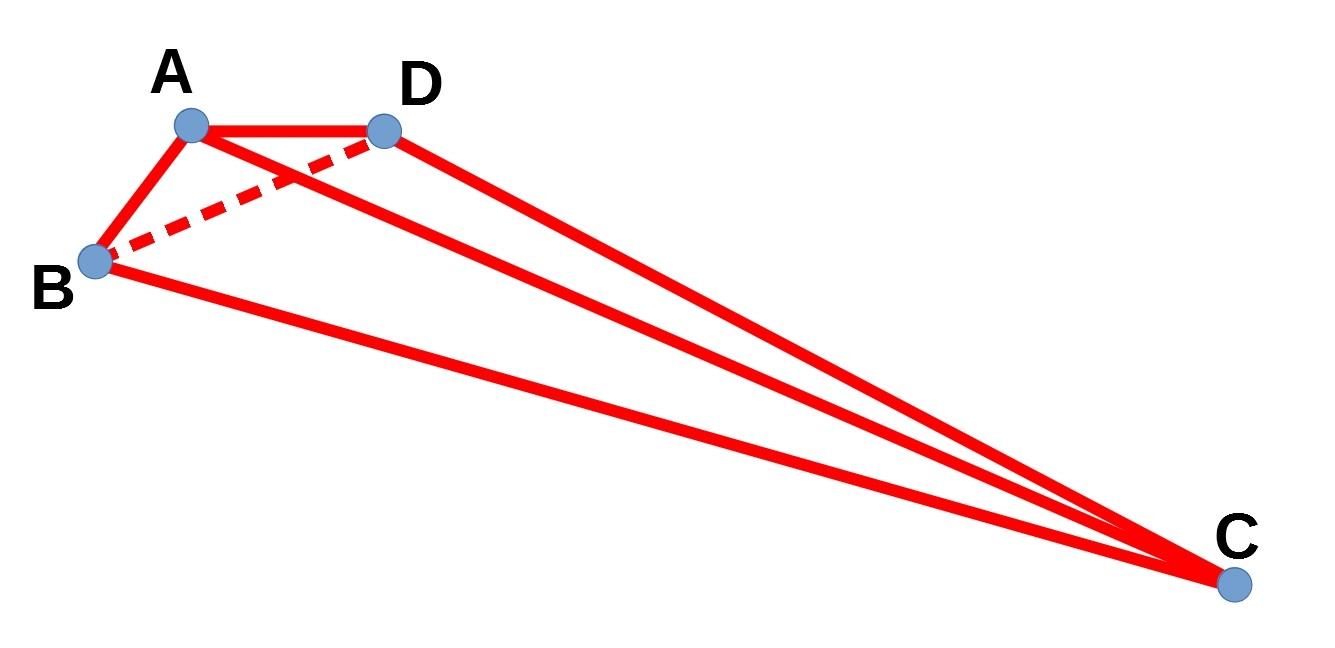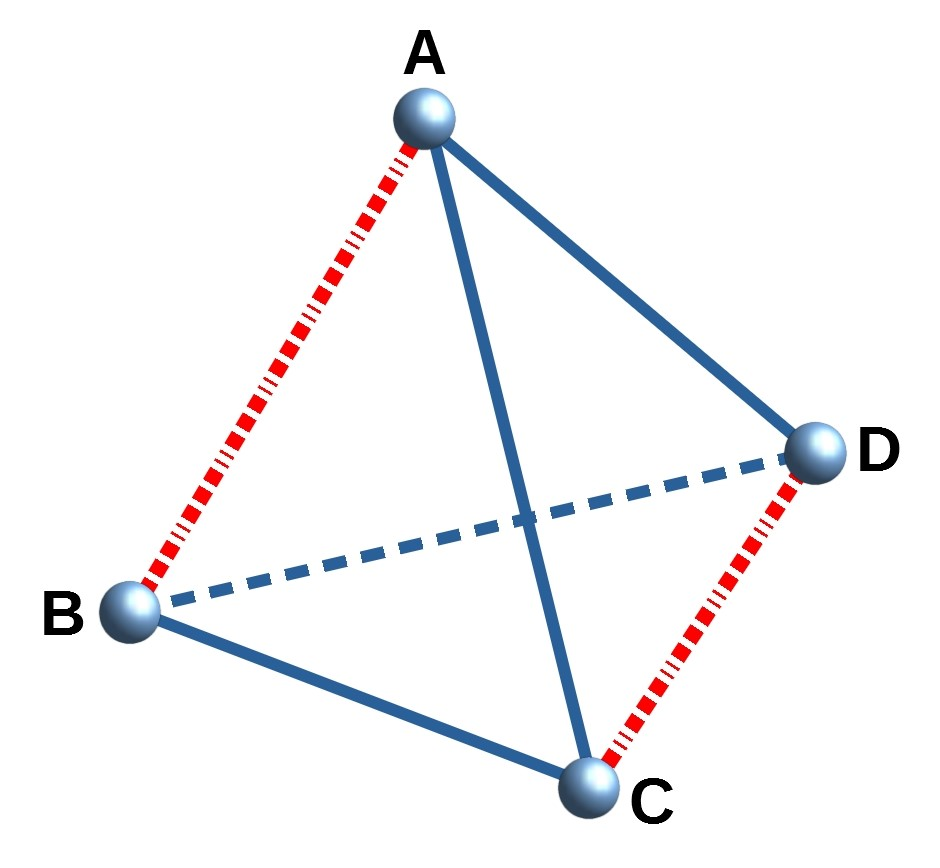#### You may also like### Shades of Fermat's Last Theorem

The familiar Pythagorean 3-4-5 triple gives one solution to (x-1)^n + x^n = (x+1)^n so what about other solutions for x an integer and n= 2, 3, 4 or 5?### Exhaustion

Find the positive integer solutions of the equation (1+1/a)(1+1/b)(1+1/c) = 2### Code to Zero

Find all 3 digit numbers such that by adding the first digit, the square of the second and the cube of the third you get the original number, for example 1 + 3^2 + 5^3 = 135.

# Tetra Inequalities

##### Age 16 to 18Challenge Level

Consider a tetrahedron $ABCD$.Can you prove that in every tetrahedron there is a vertex where the three edges meeting at that vertex have lengths which could be the lengths of the sides of a triangle?

Have a think about the problem first, and then below there are some hints and suggestions that might be useful.

---------------------------------------------------------------------------

What does this problem mean?  Can you rewrite it in a different way?

If we consider the point $A$, then there are three edges that meet this, $AB$, $AC$ and $AD$.  These three lengths might, or might not, be possible lengths of the sides of a triangle.

The problem is asking you to prove that in every tetrahedron there exists at least one vertex where the three edges meeting this vertex have lengths which could be the lengths of the sides of a triangle.

Alternatively, this problem is asking you to show that it is impossible to have a tetrahedron where none of the vertices have three edges whose lengths cannot be the lengths of the sides of a triangle.

You could try sketching some tetrahedrons to get a "feel" for the problem.

What does it mean that three lengths can be the sides of a triangle?

Which of the following sets of lengths could be the lengths of the sides of a triangle?

1. 5, 5, 8
2. 5, 5, 12
3. 3, 4, 5
4. 3, 4, 7

If a triangle has lengths $a, b, c$ where $a \le b \le c$, can you write down an inequality connecting $a, b$ and $c$?

If the three lengths can form a triangle, then the longest one must be less than the sum of the two shorter sides. We can write this as $a+b > c$.  In the case $a+b=c$ then the sides could be thought of forming a triangle with zero area, but for the purposes of this problem we will consider this to not be a triangle.

Note that we are not being asked to show that every vertex in a tetrahedron has three edges meeting it which could be the sides of a triangle.  Can you sketch out a tetrahedron where one vertex has three edges meeting it which are not a possible sets of sides of a triangle?  Click the button below to see our attempt:In this tetrahedron we have $|AB|+|BD|<|BC|$, so the three edges meeting at $B$ cannot be the sides of a triangle.  However, it does look like the three edges meeting at $C$ could be the sides of a triangle.

To try and prove the statement, we can start by assuming that there exists a tetrahedron in which none of the vertices has three edges which could be sides of a triangle. We can then try and show that this leads to a contradiction (or in other words, that this tetrahedron is impossible).

WLOG (Without Loss of Generality) let the longest edge of the tetrahedron be the edge $AB$.  (It doesn't really matter which side the longest edge is, and we can relabel the vertices so that $AB$ is the longest).  Here are a few questions to consider, bearing in mind that we have assumed that in our tetrahedron there is no vertex where the three edges that meet it have lengths which could be the sides of a triangle.

1. Can all of the six edges be the same length?
2. Can five of the edges be the same and then the last one is smaller?
3. Can four/three/two lengths be joint longest?
If all of the lengths are the same then all three edges meeting any vertex are the same, and so can form an equilateral triangle.

If three of more edges are "joint longest" then there will be at least one vertex where two of these edges meet.  Then these two edges and the third form an isosceles triangle.

It is possible to have two "joint longest" edges, as shown in the diagram below:Assuming that $AB$ is the longest side (we might have $AB=CD$), and that the three edges meeting at $A$ have lengths which cannot be the sides of a triangle, can you write down an inequality for the lengths of these edges?  Can you write down an inequality involving the three edges meeting at $B$?

If the three lengths do not form a triangle then we have $AB \ge AC + AD$.

Noting that the face $ABC$ is a triangle, can you write down an inequality involving these three edges?  Can you find a similar inequality for face $ABD$?

Can you arrange the two pairs of inequalities in such a way that they contradict each other?

You should find that you have two inequalities which for a contradiction.  This means that the original assumption that there exists a tetrahedron in which none of the vertices has three edges which could be sides of a triangle.  Therefore every tetrahedron has three edges meeting at a vertex whose lengths could form the sides of a triangle.